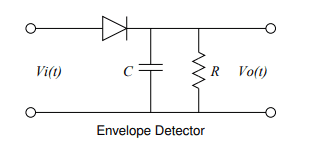# Calculate R and C in Envelope Detector Circuit (AM Modulation)

• Engineering
lottotlyl
Homework Statement:
Calculate the values for R and C on the envelope detector given below. Explain the role of the diode, resistor, and capacitor on the demodulation of the AM signal.
Relevant Equations:
Zc = -j/wC, R=V/I1. Add impedance in parallel of capacitor and resistor
• Z_rc = 1/((-wC/j) + 1/R) = (jR)/(j-wRC)
2. Ohm's law for relationship of i_d, Z_rc, and Vo
• Vo = i_d * (jR)/(j-wRC)
Unsure how to do the rest?
Thanks.

Homework Helper
Gold Member
What are input carrier and modulation frequencies?

lottotlyl
I think for this problem we are meant to assume w_c and w_m . Very theoretical.

Homework Helper
Gold Member
I think for this problem we are meant to assume w_c and w_m . Very theoretical.
OK.
Basic idea: the time costant should be large enough to smooth out the output as much as possible.
BUT - at the same time it must be small enough to see variations in the carrier amplitude if that amplitude is varied by the modulation signal.
So there's a tradeoff here. How about picking the time constant such that the modulaton amplitude is down 3dB at ## \omega_m ##? What would be the ripple output voltage given ## \omega_c ##?